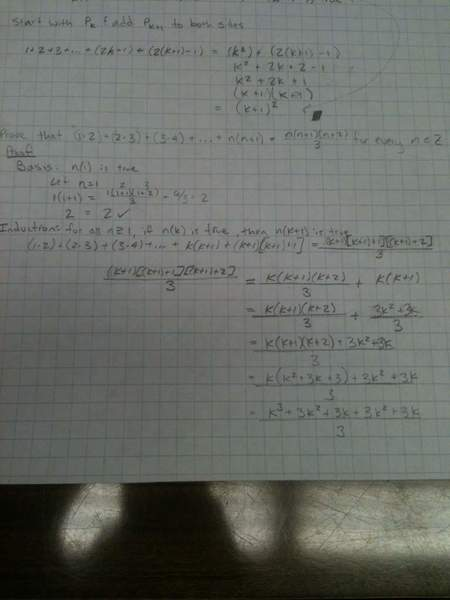# Please check my proof (in progress)

jhudson1

## Homework Statement

Prove that (1*2) + (2*3) + (3*4) + ... + n(n+1) = $$\frac{n(n+1)(n+2)}{3}$$ for every positive integer n

**edit: using mathematical induction**

## Homework Equations

In section 3 I have posted my work so far. the k^3 term is bugging me, I'm not sure how this is going to play out and if I made a mistake. I have two questions:

1) Is my notation alright? Does it look like a proof should look?
2) Can I alter the left hand side of the proof and make it match the right hand side, or should I only be working on matching the right hand side to the left hand side?

Thanks

3. Attempted Solution

#### Attachments

•induction.jpg
20.9 KB · Views: 372
Last edited:

Homework Helper
You should expand both sides and see if they match. But they won't. There is a problem with the term you are adding to the right hand side. Could you take another look at it and see if you can see what's wrong with it?

Staff Emeritus
Homework Helper
Gold Member

## Homework Statement

Prove that (1*2) + (2*3) + (3*4) + ... + n(n+1) = $$\frac{n(n+1)(n+2)}{3}$$ for every positive integer n

**edit: using mathematical induction**

## Homework Equations

In section 3 I have posted my work so far. the k^3 term is bugging me, I'm not sure how this is going to play out and if I made a mistake. I have two questions:

1) Is my notation alright? Does it look like a proof should look?
2) Can I alter the left hand side of the proof and make it match the right hand side, or should I only be working on matching the right hand side to the left hand side?

Thanks

3. Attempted Solution
That copy of your work is hard to follow, also hard to read. Here it is below/Basically for the induction step you need to prove that
$\displaystyle (1\cdot2) + (2\cdot3) + (3\cdot4) + ... + k(k+1)+(k+1)(k+2)=\frac{(k+1)(k+2)(k+3)}{3}$​
follows from assuming that
$\displaystyle (1\cdot2) + (2\cdot3) + (3\cdot4) + ... + k(k+1)=\frac{k(k+1)(k+2)}{3}$​
is true.

It doesn't look to me that this is what you've done.

With that assumption, add (k+1)(k+2) to both sides. See what you can work out.

jhudson1
Yes, I'll do that right now

jhudson1
Just worked the problem again and had a similar issue. SammyS I just now see your response. I will try it once more tonight and if I can't figure it out I'll ask my professor tomorrow.

It seems like $$k(k+1) = \frac{k(k+1)(k+2)}{3}$$

means

$$k(k+1)+(k+1)((k+1)+1) = \frac{k(k+1)(k+2)}{3}+(k+1)((k+1)+1)$$

but whenever I try to expand it I end up with a cubed term on the right hand side that I can't account for on the left hand side. Maybe it's an algebraic mistake? (Maybe not!)

I'll post another version tonight or tomorrow and hopefully it will be easier for you to follow. I'm interested in making my mathematical writing more legible for sure.

Last edited:
Homework Helper
Your statement of induction was almost right. You had (k+1)(k+2)(k+3)/3=k(k+1)(k+2)/3+k(k+1). Replace the last term k(k+1) with (k+1)(k+2) and you should have it. You see why you have to make that change, right?

Staff Emeritus
It seems like $$k(k+1) = \frac{k(k+1)(k+2)}{3}$$
$\displaystyle k(k+1) \ne \frac{k(k+1)(k+2)}{3}$
$\displaystyle (1\cdot2) + (2\cdot3) + (3\cdot4) + ... + k(k+1)=\frac{k(k+1)(k+2)}{3}$
All you need to show is that $\displaystyle \frac{k(k+1)(k+2)}{3}+(k+1)(k+2)=\frac{(k+1)(k+2)(k+3)}{3}$